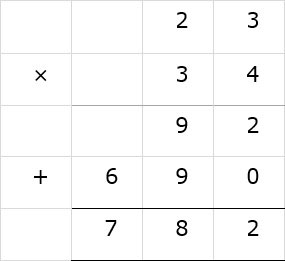# Multiplication of 2-digit numbers with 2-digit numbers

#### Complete Python Prime Pack

9 Courses     2 eBooks

#### Artificial Intelligence & Machine Learning Prime Pack

6 Courses     1 eBooks

#### Java Prime Pack

9 Courses     2 eBooks

Multiply 23 × 34

### Solution

Step 1:

First we multiply 3 of 23 with 4 of 34; 4 × 3 = 12. We bring down 2 and carry 1 to tens column.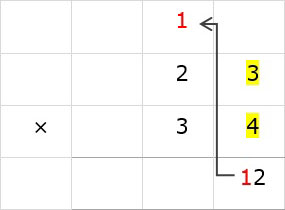Step 2:

Next we multiply 2 of 23 with 4 of 34, 4 × 2 = 8. 8 + 1 = 9. We bring down 9.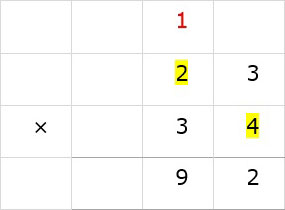Step 3:

Before further multiplication, we put a zero below 2 in ones column.

We multiply 3 of 34 with 3 of 23, 3 × 3 = 9. We bring down 9 to the left of 0.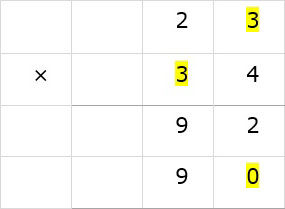Step 4:

Next we multiply 2 of 23 with 3 of 34; 3 × 2 = 6. We bring down 6.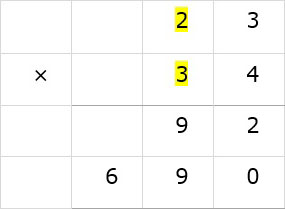Step 5:

We add up the two rows and the answer is 782.